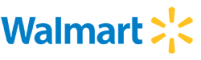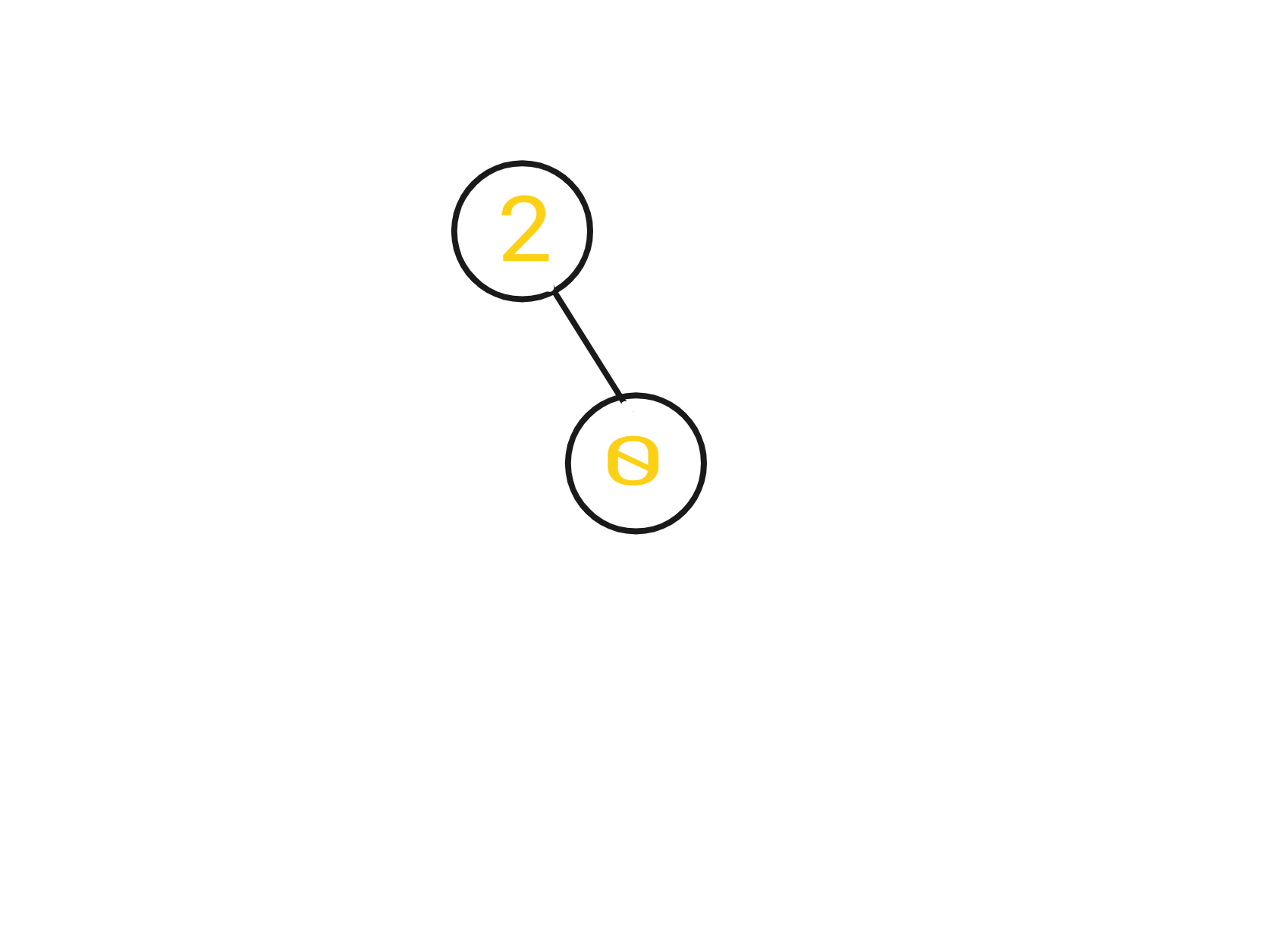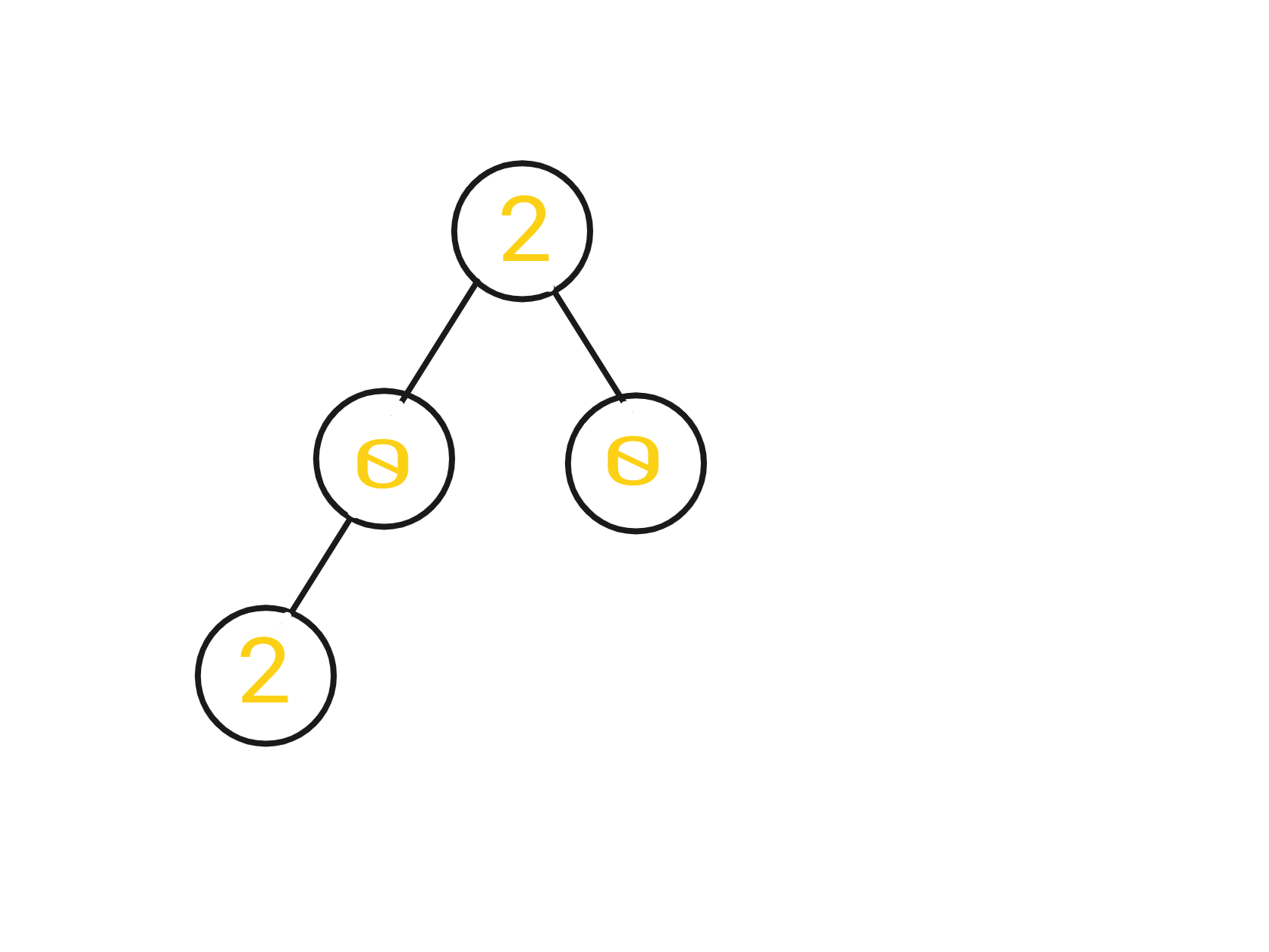New update is available. Click here to update.
Topics

# Distributing Coins

Moderate0/80
Average time to solve is 45m+3 more companies

## Problem statement

You are given the ‘ROOT’ of a binary tree with ‘N’ nodes where each node in the tree has some coins, and there are ‘N’ coins total. In one move, we may choose two adjacent nodes and move one coin from one node to another.

Your task is to return the number of moves required to make every node have exactly one coin.

Note:

``````A move may be from parent to child or from child to parent.
``````

For example,

``````Given ‘ROOT’ = [2,-1,0,-1,-1]

The tree would look like this :
````````````The answer would be 1, because the root node will transfer 1 coin to its right child. Thus both nodes have the same number of coins now.
``````
Detailed explanation ( Input/output format, Notes, Images )

#### Sample Input 1:

``````2
2 -1 0 -1 -1
2 0 2 -1 -1 -1 0 -1 -1
``````

#### Sample Output 1:

``````1
2
``````

#### Explanation of the Sample Input 1:

``````For the first test case:
The tree would look like this :
````````````The answer would be 1 because the root node will transfer 1 coin to its right child. Thus both nodes have the same number of coins now.

For the second test case:
The tree would look like this :
````````````Therefore the root node will transfer 1 coin to its right child and the leaf node will transfer 1 coin to its parent node, hence each node will have 1 coin, and the total moves made will be 2.
``````

#### Sample Input 2:

``````2
3 0 -1 -1 0 -1 -1
1 0 3 -1 -1 -1 0 -1 -1
``````

#### Sample Output 2:

``````2
4
``````Console